# 4 Equation Practice Exercises

Below are a few commands to practice typesetting. To get started, create a book or make a private chapter in an existing book for practice and make sure that the QuickLaTeX plugin is activated (see the start of the section for instructions). Solutions are in the following section.

1. A circle of radius 1: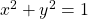2. Period of a simple harmonic oscillator: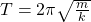3. The gravitational force between two masses:4. The solution to Basel problem: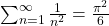Hint: The symbols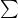is written \sum and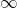is written \infty.
5. Determine what the error was in the equation for the quadratic formula shown at the end of the last section.